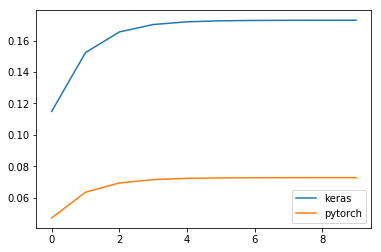# Difference in result between PyTorch and Keras when using a recurrent layer (GRU & LSTM))

Hello everyone,

I’m Léo, Ph.D. Student in deep learning, and my first post in this forum is to ask a question that has already been asked several times. However, none of the answers could solve my problem.

I’m well aware that the implementation of a GRU layer differs between Keras and Pytorch, but I’m surprised that it changes that much. I start using PyTorch a few weeks ago, and I’m maybe doing something wrong. Can you spot my mistakes?

To make it short:
when inferring a given tensor to GRU layer in Keras and PyTorch, the result is entirely different.
tensor = [1,2,3,4,5,6,7,8,9,10]To make it long:
You can find below two codes allowing you to reproduce the results of a 1-cell GRU with Keras and the same GRU with PyTorch.

• the activation and recurrent activation functions are identical for both frameworks,
• All weights are initialized to 1 to remove any difference that might be due to random initialization.
• The seed is fixed so two successive runs will always give the same result.

Keras

``````import keras.backend as K
import tensorflow as tf
import keras.layers as kl
import keras.models as km
from tensorflow import set_random_seed

import numpy as np
import matplotlib.pyplot as plt

K.clear_session()
k_t = np.array([1,2,3,4,5,6,7,8,9,10]).reshape(1, 10, 1)

# Set the seed for reproductability
np.random.seed(12345)
set_random_seed(12345)

# Create minimal keras mbodel
inp = kl.Input((10,1))
rnn = kl.GRU(1, return_sequences=True, activation='tanh', recurrent_activation='sigmoid', kernel_initializer="ones", recurrent_initializer="ones", bias_initializer="ones")(inp)
model = km.Model(input=inp, output=rnn)

keras_result = model.predict(k_t)

plt.plot(keras_result.squeeze())
plt.title("%.4f <> %.4f" % (keras_result.mean(), keras_result.std()))
plt.show()
``````

PyTorch

``````import torch
import numpy as np
import matplotlib.pyplot as plt

t_t = torch.from_numpy(np.array([1,2,3,4,5,6,7,8,9,10]).reshape(1, 10, 1)).float()

# Set the seed for reproductability
np.random.seed(12345)
torch.manual_seed(12345)

rnn = torch.nn.GRU(input_size=1, hidden_size=1, num_layers=1, batch_first=True, bidirectional=False)

# Initialization (so it is identical to keras)
for name, param in rnn.named_parameters():
if 'bias' in name:
torch.nn.init.ones_(param)
elif 'weight_ih' in name:
torch.nn.init.ones_(param)
elif 'weight_hh' in name:
torch.nn.init.ones_(param)

pytorch_result, _ = rnn(t_t)
pytorch_result = pytorch_result.detach().numpy()

plt.plot(pytorch_result.squeeze())
plt.title("%.4f <> %.4f" % (pytorch_result.mean(), pytorch_result.std()))
plt.show()

``````

Sorry for the long post, I’m really stuck here.

I’m not sure, how the `GRU` was implemented in Keras, but if I try to print `model.weights`, I only see a single bias variable. Unfortunately, I don’t know, which bias it is, as no names are printed.

However, it seems `bias_hh` might be missing, since setting it to a zero tensor, yields approx. the same results:

``````
# Initialization (so it is identical to keras)
for name, param in rnn.named_parameters():
if 'bias_ih' in name:
torch.nn.init.ones_(param)
elif 'bias_hh' in name:
torch.nn.init.zeros_(param)
elif 'weight_ih' in name:
torch.nn.init.ones_(param)
elif 'weight_hh' in name:
torch.nn.init.ones_(param)

> array([[0.11491495],
[0.15235901],
[0.16547596],
[0.170214  ],
[0.17194527],
[0.1725806 ],
[0.17281413],
[0.17290002],
[0.17293155],
[0.17294317]], dtype=float32)
``````

Hello ptrblck,
So sorry to answer that late. I was busy with something different for my Ph.D. I just try it out, and the result is identical. Thanks so much.

I will try this out in my real model now and check if I manage to get the same results than I had with Keras.

Thanks again !

Were you able to get the same results? I’m actually struggling with the same thing